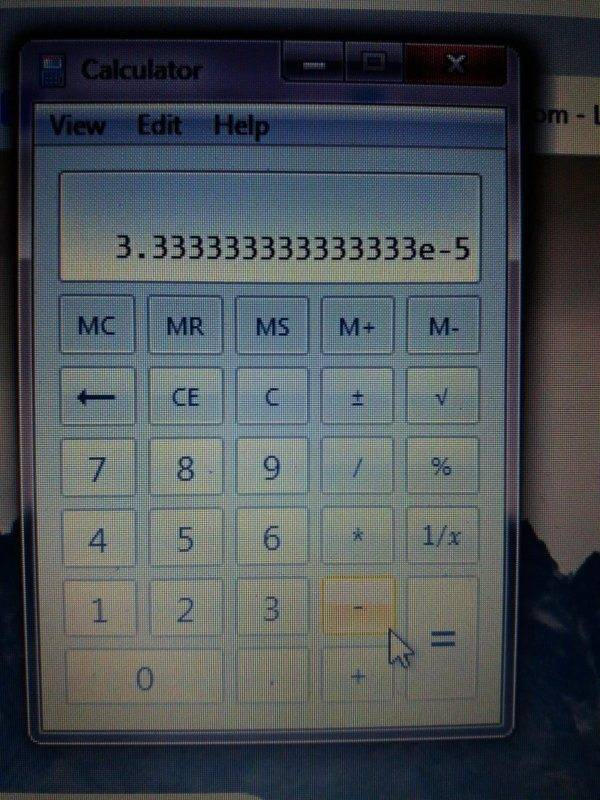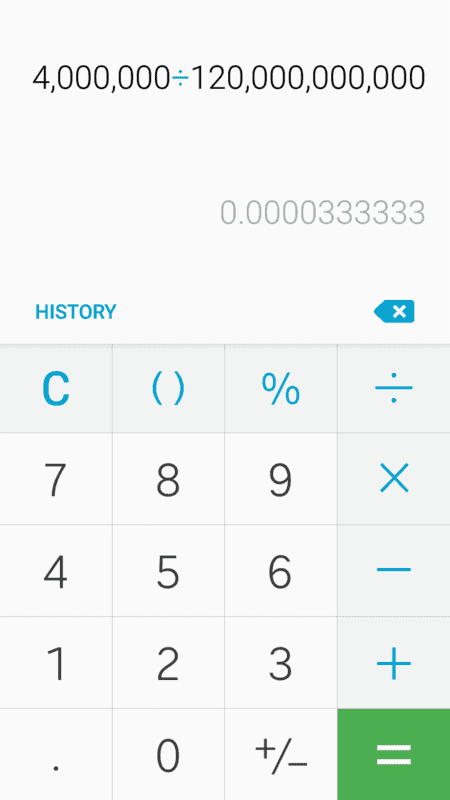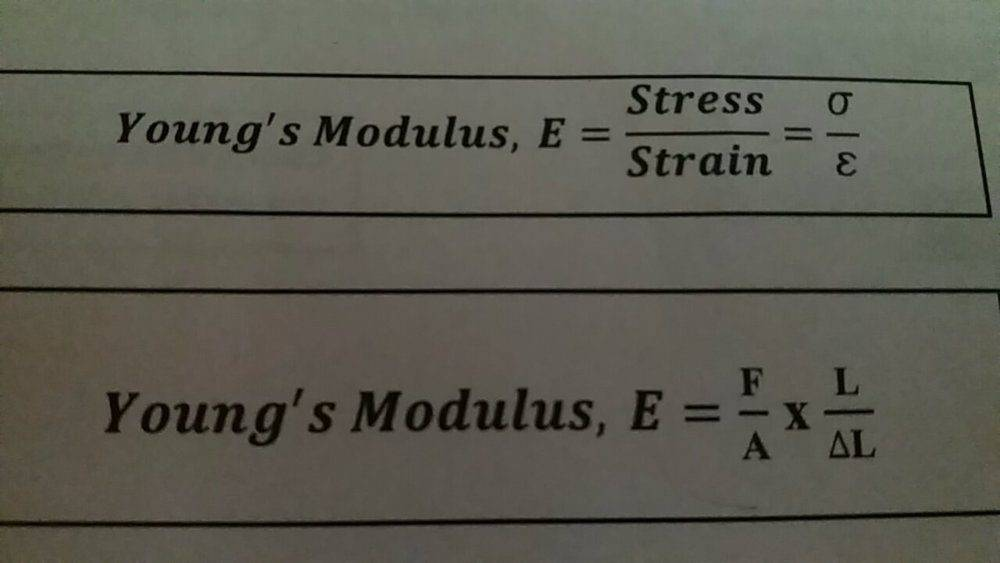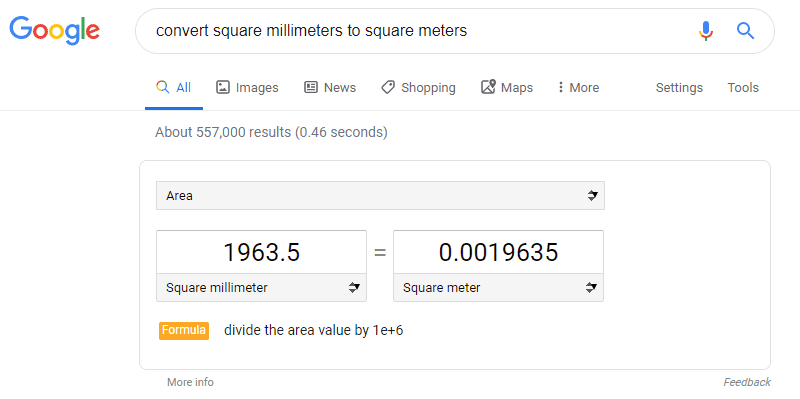# Unit conversions involving Pascals

• chriscarson
I will need a very basic lesson to understand this .No problem! There's plenty of resources online to get you started. Take a look at some of these links:https://math.stackexchange.com/questions/514070/what-does-the-exponentiation-of-negative-numbers-meanhttps://math.stackexchange.com/questions/214553/what-is-the-meaning-of-the-exponentiation-of-negative-numbersf
Ok . thanks again
Note the caveats I've edited into the previous post.

•chriscarson
Yes, almost certainly. The units I would expect area to be reported in would be square meters.

However... The rules for significant figures suggest that less precision should be reported. And the calculated result you give is incorrect in the last two digits.

I did , 0.025m x 0.025m = 0.000625 m2

0.000625 x 3.142 = 0.00196375m2

Last edited:
I did , 0.025m x 0.025m = 0.000625 m2

0.000625 x 3.142 = 0.00196375m2
##\pi## is not equal to 3.142 -- at least not to the six significant figures that you quoted in the result.

•chriscarson
##\pi## is not equal to 3.142 -- at least not to the six significant figures that you quoted in the result.

so that could be were I m mistaken ?

##\pi## is not equal to 3.142 -- at least not to the six significant figures that you quoted in the result.

so that could be were I m mistaken ?

so that could be were I m mistaken ?
You've not told us that someone told you that you were mistaken. We are not mind readers.

You've not told us that someone told you that you were mistaken. We are not mind readers.

I mean I am asking if it is that what I am doing wrong. If it is because I am using only till 3.142 than 6 significant figures.

I mean I am asking if it is that what I am doing wrong. If it is because I am using only till 3.142 than 6 significant figures.
When in doubt, check it out.

Let us make three attempts at the calculation. One using the truncated figure for ##\pi##, one using a value of pi that is good for six figures and one using a value that is good for as much as Windows calculator will do.

##\pi## is approximately 3.141_592_653_589_793_238_462_6. Usually, people remember it to three digits (3.14) because the next digit is a one and it's a no brainer to round 3.141 down to 3.14. Often people remember it to 5 digits (3.1416) because the next digit is a nine and it's a no brainer to round 3.14159 up to 3.1416. However, you have chosen to remember it to 4 digits (3.142), rounding 3.1415 up to 3.142. That's a remarkably poor place to truncate the decimal expansion since it was nearly a 50/50 choice to round up or down, given that the first truncated digit was a five.

On to the checking out part...

Four digit calculation: 3.142 x 0.0252: Calculator says 0.00196375. Rounded to six significant figures, that is still 0.00196375. Rounded to two significant figures, it is 0.0020.

Six digit calculation: 3.14159 x 0.0252: Calculator says 0.00196349375. Rounded to six significant figures, that is 0.00196349. Rounded to two significant figures, it is 0.0020.

My windows calculator limit: 3.1415926535897932384626433832795 x 0.0252: Calculator says 0.00196349540849362077403915211455. Rounded to six significant figures, that is 0.00196350. Rounded to two significant figures, it is 0.0020.

Note how the last two digits in the six figure result changed depending on the accuracy of the figure used for pi. Note how the last digit in the six figure result was incorrect even though the calculation used a value for pi that was good to six digits.

1. If you are going to use a defined constant, use it with all available precision.
2. If you are going to compute an intermediate result, use all available precision.
3. When you report a final result, round it to the appropriate number of significant figures. In this case, with inputs good to two significant figures, the result should have been reported to two digits or certainly no more than three.

These rules of thumb will get you through at least freshman physics. Real numerical analysis and error analysis goes way deeper.

Last edited:
•chriscarson
thanks for your time I will study more to what you wrote , it will help for sure.

So from you wrote , the best thing is that they tell you to how many numbers should be rounded , the pi and the answer.

When you make 3.14 x ( 0.025m x 0.025m) = 0.0019625m2 converted to mm is 1.9625mm2

and if you make 3.14 x ( 25mm x 25mm ) = 1962.5mm2 converted to m is 1.9625m2

I think it s a matter when you convert or in the question or in the answer . Results are different.

When you make 3.14 x ( 0.025m x 0.025m) = 0.0019625m2 converted to mm is 1.9625mm2
No, it is not.

As I'd already pointed out in post #27, the conversion factor between square meters and square millimeters is 1,000,000. There are one million squares, one millimeter on a side in a square that is one meter on a side.

You are not converting from meters to millimeters. You are converting from square meters to square millimeters.

$$0.0019625 \text{ m}^2 \times \frac{1,000,000 \text{ mm}^2}{1 \text{ m}^2} = 1962.5 \text{ mm}^2$$

I am running out of different ways to say this.

•chriscarson
No, it is not.

As I'd already pointed out in post #27, the conversion factor between square meters and square millimeters is 1,000,000. There are one million squares, one millimeter on a side in a square that is one meter on a side.

You are not converting from meters to millimeters. You are converting from square meters to square millimeters.

$$0.0019625 \text{ m}^2 \times \frac{1,000,000 \text{ mm}^2}{1 \text{ m}^2} = 1962.5 \text{ mm}^2$$

oh I think I get it now so the best is convert in the question , because they told me that the answer have to be in Newtons and meters.

oh I think I get it now so the best is convert in the question , because they told me that the answer have to be in Newtons and meters.
Given an input measurement of 25 millimeters radius and desiring a result for the area of a corresponding circle, there are two ways to proceed.

1. Convert 25 millimeters to meters and compute ##\pi r^2## with the result in square meters.
2. Compute ##\pi r^2## with the result in square millimeters and convert the result to square meters.

Let us proceed with the first approach.
$$r=25\text{ mm} \times \frac{1\text{ m}}{1000\text{ mm}}=0.025\text{m}$$ $$a=\pi r^2=\pi (0.025\text{ m})^2=0.0019635\text{ m}^2$$ Round to 0.0020 m2

Let us try the second approach instead.
$$r=25\text{ mm}$$ $$a=\pi r^2=\pi\ (25\text{ mm})^2=1963.5\text{ mm}^2=1963.5\text{ mm}^2 \times (\frac{1\text{ m}}{1000\text{ mm}})^2=\frac{1963.5}{1000 \times 1000}\text{ m}^2 = 0.0019635\text{ m}^2$$ Round to 0.0020 m2

Six of one. Half dozen of the other.

Last edited:
•chriscarson
because they told me that the answer have to be in Newtons and meters.
Who is "they"? Has this been for your homework all along?

Who is "they"? Has this been for your homework all along?

The teacher said you have always to give the result in N/m2 (Newton meter squared)

Given an input measurement of 25 millimeters radius and desiring a result for the area of a corresponding circle, there are two ways to proceed.

1. Convert 25 millimeters to meters and compute ##\pi r^2## with the result in square meters.
2. Compute ##\pi r^2## with the result in square millimeters and convert the result to square meters.

Let us proceed with the first approach.
$$r=25\text{ mm} \times \frac{1\text{ m}}{1000\text{ mm}}=0.025\text{m}$$ $$a=\pi r^2=\pi (0.025\text{ m})^2=0.0019635\text{ m}^2$$ Round to 0.0020 m2

Let us try the second approach instead.
$$r=25\text{ mm}$$ $$a=\pi r^2=\pi\ (25\text{ mm})^2=1963.5\text{ mm}^2=1963.5\text{ mm}^2 \times (\frac{1\text{ m}}{1000\text{ mm}})^2=\frac{1963.5}{1000 \times 1000}\text{ m}^2 = 0.0019635\text{ m}^2$$ Round to 0.0020 m2

Six of one. Half dozen of the other.

Ok so you did convert from square mm to square m but in the proper way , I used an online converter.I always stop to something when I try to make a sum , this time I have this to make me confuse , same exactly sum but different calculators different results .

That's the same result, just written in a different format. The e-5 at the end of the first number is a standard computer and calculator way of writing ##×10^{-5}##. So that number is ##3.333×10^{-5}##, which is ##3.333×\frac 1{10^5}## or ##3.333×\frac 1{100,000}## or 0.00003333, which is what the other calculator says.

•chriscarson
That's the same result, just written in a different format. The e-5 at the end of the first number is a standard computer and calculator way of writing ##×10^{-5}##. So that number is ##3.333×10^{-5}##, which is ##3.333×\frac 1{10^5}## or ##3.333×\frac 1{100,000}## or 0.00003333, which is what the other calculator says.

Oh so much to learn I guess .
Thanks

Oh so much to learn I guess .
Thanks
This stuff is like learning where to put your fingers on a guitar or something. It's slow going and you get a lot of nasty sounds, but you have to learn it if you want to play. It does eventually become second nature and it's really difficult to remember not knowing how to do it.•chriscarson
This stuff is like learning where to put your fingers on a guitar or something. It's slow going and you get a lot of nasty sounds, but you have to learn it if you want to play. It does eventually become second nature and it's really difficult to remember not knowing how to do it.great simplification of what you mean.going to keep this quote to motivate who s going to give up, well done

Last edited:
And it keeps going with strange things, last question is to find the young modulus , in the notes if found this to confuse me , E is equal to two different formulasE is equal to two different formulas
Use the one for which you have the required variables.

•chriscarson
Note that the ##x## in the second formula should be the multiplication symbol ×.

Stress is force over area. Strain is length change over original length. The second formula is what you get when you substitute those definitions into the first formula.

•Ok so you did convert from square mm to square m but in the proper way , I used an online converter.
Online converters work. Just have to use the right units. I Googled "convert square millimeters to square meters". Without leaving the Google page, a converter was displayed. I keyed in 1963.5.•chriscarson
Online converters work. Just have to use the right units. I Googled "convert square millimeters to square meters". Without leaving the Google page, a converter was displayed. I keyed in 1963.5.
View attachment 255555

Good nit saying that it s an area value it was a mistake

Use the one for which you have the required variables.

Yeah and is it that you multiply first or work the division separated and the multiply the results ?

Yeah and is it that you multiply first or work the division separated and the multiply the results ?
Multiply what by what or work what division? Are you seriously asking how to evaluate ##\frac{a}{b} \times \frac{c}{d}##?

Multiply what by what or work what division? Are you seriously asking how to evaluate ##\frac{a}{b} \times \frac{c}{d}##?

Yes is that so basic ?

Yes is that so basic ?
Here in the U.S. we are taught to add and multiply fractions at about age eight.

For ##\frac{a}{b} \times \frac{c}{d}## there are many ways to evaluate the result and get the same right answer. The canonical "right" way is to:

1. Divide a by b giving the result ##\frac{a}{b}##.
2. Divide c by d giving the result ##\frac{c}{d}##.
3. Multiply those two results together.

Another way is to:

1. Multiply a by c giving the result ##ac##.
2. Multiply b by d giving the result ##bd##.
3. Divide the result from 1) by the result from 2).

It is a rule of arithmetic and of real algebra that$$\frac{a}{b} \times \frac{c}{d} = \frac{ac}{bd}$$

One rule of algebra that they didn't teach us until age 16 or so was that putting two variable names side by side ("juxtaposition") is a notation that conventionally means "multiply them together".

[As it turns out my first exposure to the juxtaposition notation was in a standardized test -- they tested on it before my school had ever presented the content. It is over 40 years later now and that incident still cheeses me off]

•Ibix and chriscarson
Here in the U.S. we are taught to add and multiply fractions at about age eight.

For ##\frac{a}{b} \times \frac{c}{d}## there are many ways to evaluate the result and get the same right answer. The canonical "right" way is to:

1. Divide a by b giving the result ##\frac{a}{b}##.
2. Divide c by d giving the result ##\frac{c}{d}##.
3. Multiply those two results together.

Another way is to:

1. Multiply a by c giving the result ##ac##.
2. Multiply b by d giving the result ##bd##.
3. Divide the result from 1) by the result from 2).

It is a rule of arithmetic and of real algebra that$$\frac{a}{b} \times \frac{c}{d} = \frac{ac}{bd}$$

One rule of algebra that they didn't teach us until age 16 or so was that putting two variable names side by side ("juxtaposition") is a notation that conventionally means "multiply them together".

[As it turns out my first exposure to the juxtaposition notation was in a standardized test -- they tested on it before my school had ever presented the content. It is over 40 years later now and that incident still cheeses me off]

Yeah finally is that what I thought but I m finding so many obstacles that I want to be 100% in every tiny thing .

•chriscarson